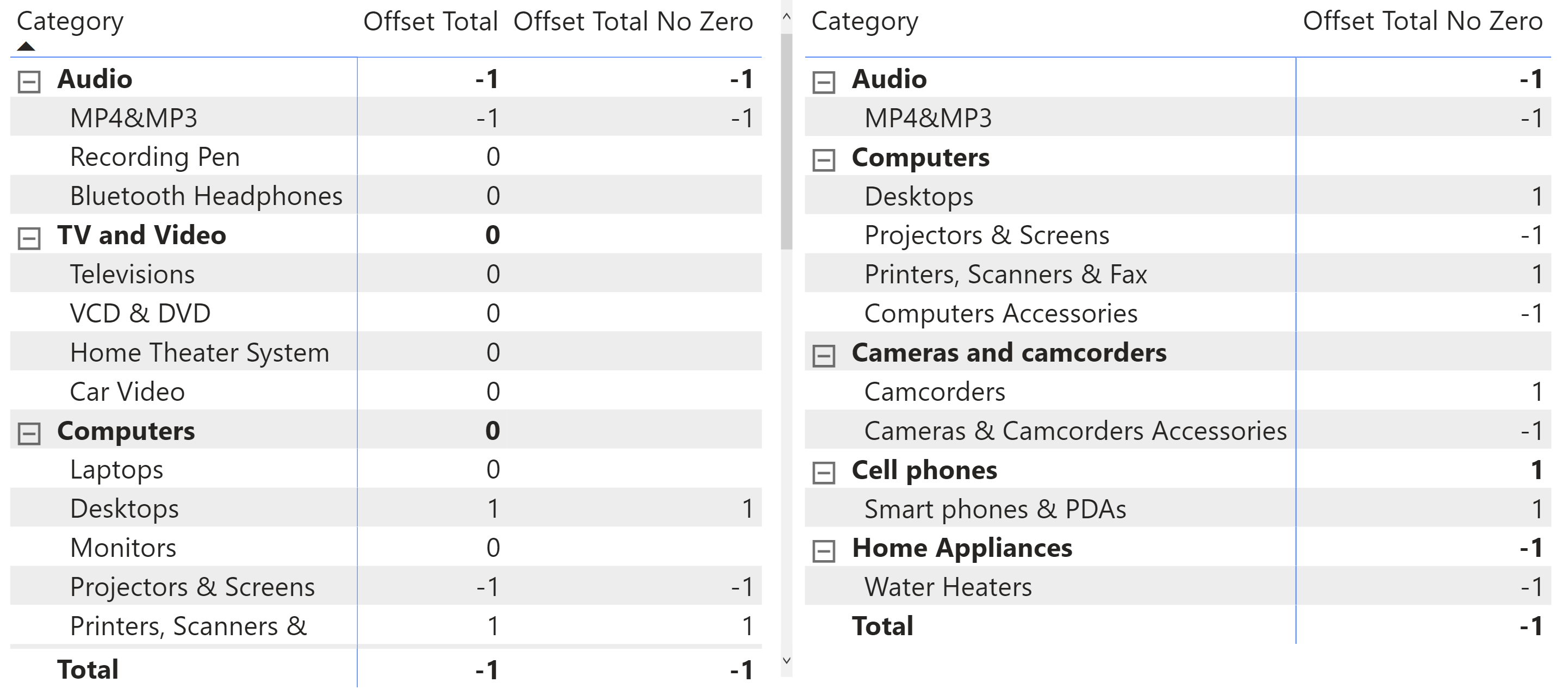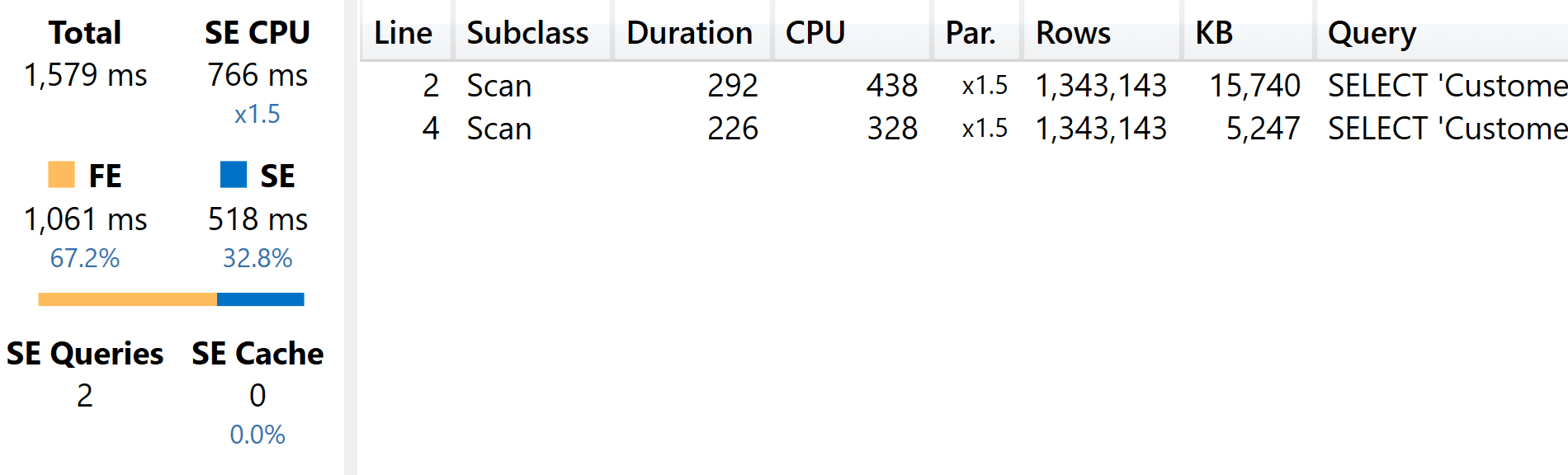In matrix visuals, Power BI usually hides rows where all the measures return a blank value. To leverage this behavior or simply to change the visualization of a measure depending on its result, you might want to achieve one of the following:

• Transforming a blank result to zero: this is covered in the article, How to return 0 instead of BLANK in DAX.
• Transforming a zero result to blank: this is the scenario described in this article.

You will typically be applying this technique to measures like a balance amount. To use a simple example, we sum the Product[Offset] column in the Offset Total measure. We obtain the result on the left-hand side of the following screenshot.The goal of the Offset Total No Zero measure is to replace “0” with a blank value so that we get the result on the right-hand side of the screenshot: only the rows with a value other than zero are being displayed in this report. We have different techniques available to us, which differ in readability and performance.

The first option is to use the IF function, as we do in the Offset Total Non Zero IF measure:

Measure in the Product table
```Offset Total No Zero IF :=
VAR SumOffset =
SUM ( 'Product'[Offset] )
RETURN
IF ( SumOffset = 0, BLANK (), SumOffset )
```

This technique produces the expected result and evaluates the sum of Customer[Offset] only once, thanks to a variable. However, the use of IF results in a suboptimal query plan. A faster option would be to use DIVIDE, as shown in the Offset Total Non Zero measure:

Measure in the Product table
```Offset Total No Zero :=
VAR SumOffset =
SUM ( 'Product'[Offset] )
RETURN
SumOffset * DIVIDE ( SumOffset, SumOffset )
```

If the value of SumOffset is multiplied by a DIVIDE of a number by itself, that means that SumOffset should be multiplied by 1. But if the arguments of DIVIDE are zero, the result of DIVIDE is blank – and this in turn propagates blank to the result of the multiplication.

While the approach using DIVIDE results in being less readable, it presents a performance advantage that could be important if a measure is evaluated in a high-cardinality iterator. This scenario includes the visualizations where the measure is evaluated potentially for millions of rows, even though only a few hundred rows are displayed: underneath, all the rows might have to be computed. This will have a visible impact on the execution of the query.

We tested performance with a similar model where we implemented the Offset calculation on a Customer table with 2 million rows. The measure is evaluated when iterating Customer[Name] – which has 1.3 million unique values – using the following DAX query:

DAX Query
```DEFINE
VAR __DS0Core =
SUMMARIZECOLUMNS (
'Customer'[Name],
"Offset_Total_No_Zero", [Offset Total No Zero]
)

EVALUATE
TOPN (
502,
__DS0Core,
[Offset_Total_No_Zero], DESC,
'Customer'[Name], ASC
)
ORDER BY
[Offset_Total_No_Zero] DESC,
'Customer'[Name]
```

Using the Offset Total No Zero IF measure instead of Offset Total No Zero in the SUMMARIZECOLUMNS function, we spend 1,061 milliseconds in the formula engine and 518 milliseconds on two storage engine queries.When using the Offset Total No Zero optimized function, we save 47% of formula engine consumption – which is reduced to 563 milliseconds – and another 38% of the storage engine because there is only one storage engine query being executed.In a way, the result is not surprising: the IF function in a measure can be expensive when the result of the predicate is different for every evaluation (in this case, for every customer). Using DIVIDE, we use an arithmetic operation with the same execution path for all the customers. DIVIDE is not as efficient as a regular division. Still, thanks to its behavior when there is a division by zero, it manages the conditional logic – returning blank when there is a division by zero – more efficiently than any other DAX function.

Thus, if the optimization is critical for your measure, consider using DIVIDE as an optimization technique for this scenario. Otherwise, the IF function produces an easier to read and to maintain code.

IFChecks whether a condition is met, and returns one value if TRUE, and another value if FALSE.

`IF ( <LogicalTest>, <ResultIfTrue> [, <ResultIfFalse>] )`

DIVIDESafe Divide function with ability to handle divide by zero case.

`DIVIDE ( <Numerator>, <Denominator> [, <AlternateResult>] )`

SUMMARIZECOLUMNSCreate a summary table for the requested totals over set of groups.

`SUMMARIZECOLUMNS ( [<GroupBy_ColumnName> [, [<FilterTable>] [, [<Name>] [, [<Expression>] [, <GroupBy_ColumnName> [, [<FilterTable>] [, [<Name>] [, [<Expression>] [, … ] ] ] ] ] ] ] ] ] )`